JEE  >  Test: Single Correct MCQs: Electrostatics | JEE Advanced

# Test: Single Correct MCQs: Electrostatics | JEE Advanced

Test Description

## 34 Questions MCQ Test Physics For JEE | Test: Single Correct MCQs: Electrostatics | JEE Advanced

Test: Single Correct MCQs: Electrostatics | JEE Advanced for JEE 2022 is part of Physics For JEE preparation. The Test: Single Correct MCQs: Electrostatics | JEE Advanced questions and answers have been prepared according to the JEE exam syllabus.The Test: Single Correct MCQs: Electrostatics | JEE Advanced MCQs are made for JEE 2022 Exam. Find important definitions, questions, notes, meanings, examples, exercises, MCQs and online tests for Test: Single Correct MCQs: Electrostatics | JEE Advanced below.
Solutions of Test: Single Correct MCQs: Electrostatics | JEE Advanced questions in English are available as part of our Physics For JEE for JEE & Test: Single Correct MCQs: Electrostatics | JEE Advanced solutions in Hindi for Physics For JEE course. Download more important topics, notes, lectures and mock test series for JEE Exam by signing up for free. Attempt Test: Single Correct MCQs: Electrostatics | JEE Advanced | 34 questions in 70 minutes | Mock test for JEE preparation | Free important questions MCQ to study Physics For JEE for JEE Exam | Download free PDF with solutions
 1 Crore+ students have signed up on EduRev. Have you?
Test: Single Correct MCQs: Electrostatics | JEE Advanced - Question 1

### A hollow metal sphere of radius 5 cms is  charged such that the potential on its surface is 10 volts. The  potential at the centre of the sphere is

Detailed Solution for Test: Single Correct MCQs: Electrostatics | JEE Advanced - Question 1

The potential at the surface of a hollow or conducting sphere is same as the potential at the centre of the sphere and any point inside the sphere.

Test: Single Correct MCQs: Electrostatics | JEE Advanced - Question 2

### Two point charges +q and –q are held fixed at (–d, o) and (d, o) respectively of a x-y coordinate system. Then

Detailed Solution for Test: Single Correct MCQs: Electrostatics | JEE Advanced - Question 2

The two charges form an electric dipole. If  we take a point M on the X-axis as shown in the figure, then the net electric field is in –X-direction.

∴ Option (a) is incorrect.
If we take a point N on Yaxis, we find net electric field along  + X direction.
The same will be true for any point on Y-axis. (b) is a correct option.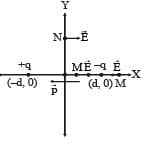NOTE : For any point on the equatorial line of a dipole, the electric field is opposite to the direction of dipole moment.

(b) W∞ – 0 = q (V–V0) = q (0 – 0) = 0
∴ (c) is incorrect. The direction of dipole moment is from –ve to + ve. Therefore (d) is incorrect.

Test: Single Correct MCQs: Electrostatics | JEE Advanced - Question 3

### A parallel plate capacitor of capacitance C is connected to a battery and is charged to a potential difference V. Another capacitor of capacitance 2C is similarly charged to a potential difference 2V. The charging battery is now disconnected and the capacitors are connected in parallel to each other in such a way that the positive terminal of one is connected to the negative terminal of the other. The final energy of the configuration is

Detailed Solution for Test: Single Correct MCQs: Electrostatics | JEE Advanced - Question 3

C and 2C are in parallel to each other.

∴ Resultant capacity = (2C + C)
CR = 3C
Net potential = 2V – V
VR = V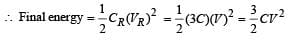Test: Single Correct MCQs: Electrostatics | JEE Advanced - Question 4

Two identical metal plates are given positive charges Q1 and Q2 (<Q1) respectively. If they are now brought close together to form a parallel plate capacitor with capacitance C, the potential difference between them is

Detailed Solution for Test: Single Correct MCQs: Electrostatics | JEE Advanced - Question 4

Within the capacitor,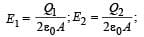where  A = area of each plate
d = separation between two plate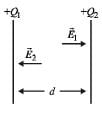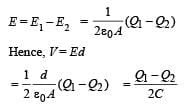Test: Single Correct MCQs: Electrostatics | JEE Advanced - Question 5

For the circuit shown in Figure, which of the following statements is true?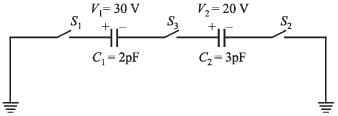Detailed Solution for Test: Single Correct MCQs: Electrostatics | JEE Advanced - Question 5

With the closing of switch  S3 and S1 the negative charge on C2 will attract the positive charge on C2 thereby maintaining the negative charge on C1. The negative charge on C1 will attract the positive charge on C1. No transfer of charge will take place. Therefore p.d across C1 and C2 will be 30 V and 20 V.

Test: Single Correct MCQs: Electrostatics | JEE Advanced - Question 6

Three charges Q, +q and +q are placed at the vertices of a right-angled isosceles triangle as shown. The net electrostatic energy of the configuration is zero if Q is equal to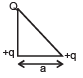Detailed Solution for Test: Single Correct MCQs: Electrostatics | JEE Advanced - Question 6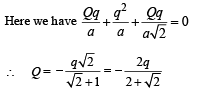Test: Single Correct MCQs: Electrostatics | JEE Advanced - Question 7

A parallel plate capacitor of area A, plate separation d and capacitance C is filled with three different dielectric materials having dielectric constants k1, k2 and k3 as shown. If a single dielectric material is to be used to have the same capacitance C in this capacitor, then its dielectric constant k is given by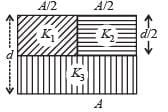Detailed Solution for Test: Single Correct MCQs: Electrostatics | JEE Advanced - Question 7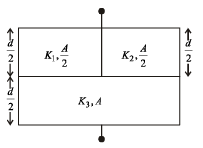Let C1 = Capacity of capacitor with K1
C2 = Capacity of capacitor with K2
C3 = Capacity of capacitor with K3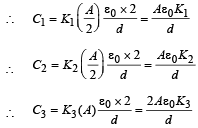C1 and C2 are in parallel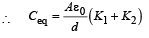Ceq and C3 are in series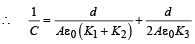But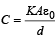for single equivalent capacitor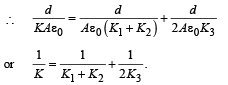Test: Single Correct MCQs: Electrostatics | JEE Advanced - Question 8

Three positive charges of equal value q are placed at the vertices of an equilateral triangle. The resulting lines of force should be sketched as in

Detailed Solution for Test: Single Correct MCQs: Electrostatics | JEE Advanced - Question 8

Electric field lines do not form closed loops. Therefore options (a), (b) and (d) are wrong.
Option (c) is correct. There is repulsion between similar charges.

Test: Single Correct MCQs: Electrostatics | JEE Advanced - Question 9

Consider the situation shown in the figure. The capacitor A has a charge q on it whereas B is uncharged. The charge appearing on the capacitor B a long time after the switch is closed is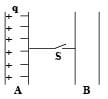Detailed Solution for Test: Single Correct MCQs: Electrostatics | JEE Advanced - Question 9

When S is closed, there will be no shifting of negative charge from plate A to B as the charge – q is held by the charge + q. Neither there will be any shifting of charge from B to A.

Test: Single Correct MCQs: Electrostatics | JEE Advanced - Question 10

A uniform electric field pointing in positive x-direction exists in a region. Let A be the origin, B be the point on the x-axis at x = +1 cm and C be the point on the y-axis at y = +1 cm. Then the potentials at the points A, B and C satisfy:

Detailed Solution for Test: Single Correct MCQs: Electrostatics | JEE Advanced - Question 10

NOTE :  As we move along the direction of electric field the potential decreases.

∴ VA > VB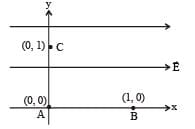Test: Single Correct MCQs: Electrostatics | JEE Advanced - Question 11

Two equal point charges are fixed at x = – a and x = + a on the x-axis. Another point charge Q is placed at the origin.
The change in the electrical potential energy of Q, when it is displaced by a small distance x along the x-axis, is approximately proportional to

Detailed Solution for Test: Single Correct MCQs: Electrostatics | JEE Advanced - Question 11

Initial energy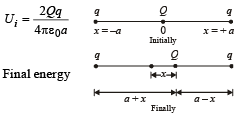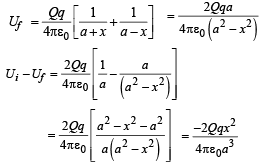when x << a then x2 is neglected in denominator..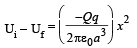Test: Single Correct MCQs: Electrostatics | JEE Advanced - Question 12

Two identical capacitors, have the same capacitance C. One of them is charged to potential V1 and the other V2. The negative ends of the capacitors are connected together.
When the positive ends are also connected, the decrease in energy of the combined system is

Detailed Solution for Test: Single Correct MCQs: Electrostatics | JEE Advanced - Question 12

Initially we know that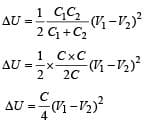Test: Single Correct MCQs: Electrostatics | JEE Advanced - Question 13

A metallic shell has a point charge ‘q’ kept inside its cavity. Which one of the following diagrams correctly represents the electric lines of forces?

Detailed Solution for Test: Single Correct MCQs: Electrostatics | JEE Advanced - Question 13

Electric field everywhere inside the metallic portion of shell is zero.
Hence options (a) and (d) are incorrect.
Electric field lines are always normal to a surface. Hence option (b) is incorrect. Only option (c) represents the correct answer.

Test: Single Correct MCQs: Electrostatics | JEE Advanced - Question 14

Six charges of equal magnitude, 3 positive and 3 negative are to be placed on PQRSTU corners of a regular hexagon, such that field at the centre is double that of what it would have been if only one +ve charge is placed at R. Which of the following arrangement of charge is possible for P, Q, R, S, T and U respectively.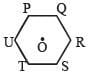Detailed Solution for Test: Single Correct MCQs: Electrostatics | JEE Advanced - Question 14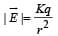Electric field due to P on O is cancelled by electric field due to S on O.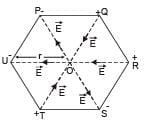to Q on O is cancelled by electric field due to T and O.
The electric field due to R on O is in the same direction as that of U on O.
Therefore the net electric field is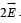Test: Single Correct MCQs: Electrostatics | JEE Advanced - Question 15

A Gaussian surface in the figur e is shown by dotted line. The electric field on the surface will be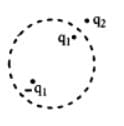Detailed Solution for Test: Single Correct MCQs: Electrostatics | JEE Advanced - Question 15

The flux through the Gaussian surface is due to the charges inside the Gaussian surface. But the electric field on the Gaussian surface will be due to the charges present in side the Gaussian surface and outside it. It will be due to all the charges.

Test: Single Correct MCQs: Electrostatics | JEE Advanced - Question 16

Three infinitely long charge sheets are placed as shown in figure. The electric field at point P is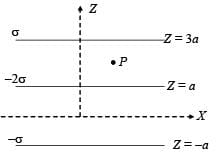Detailed Solution for Test: Single Correct MCQs: Electrostatics | JEE Advanced - Question 16

Figure shows the electric fields due to the sheets 1, 2 and 3 at point P. The direction of electric fields are according to the charge on the sheets (away from positively charge sheet and towards the negatively charged sheet and perpendicular).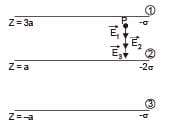The total electric field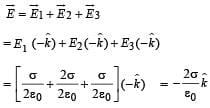Test: Single Correct MCQs: Electrostatics | JEE Advanced - Question 17

A long, hollow conducting cylinder is kept coaxially inside another long, hollow conducting cylinder of larger radius.
Both the cylinders are initially electrically neutral.

Detailed Solution for Test: Single Correct MCQs: Electrostatics | JEE Advanced - Question 17

When a charge density is given to the inner cylinder, the potential developed at its surface is different from that on the outer cylinder. This is because the potential decreases with distance for a charged conducting cylinder when the point of consideration is outside the cylinder.
But when a charge density is given to the outer cylinder, it will change its potential by the same amount as that of the inner cylinder. Therefore no potential difference will be produced between the cylinders in this case.

Test: Single Correct MCQs: Electrostatics | JEE Advanced - Question 18

Consider a neutral conducting sphere. A positive point charge is placed outside the sphere. The net charge on the sphere is then

Detailed Solution for Test: Single Correct MCQs: Electrostatics | JEE Advanced - Question 18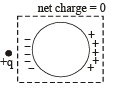When a positive point charge is placed outside a conducting sphere, a rearrangement of charge takes place on the surface.
But the total charge on the sphere is zero as no charge has left or entered the sphere.

Test: Single Correct MCQs: Electrostatics | JEE Advanced - Question 19

A spherical portion has been removed from a solid sphere having a charge distributed uniformly in its volume as shown in the figure. The electric field inside the emptied space is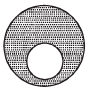Detailed Solution for Test: Single Correct MCQs: Electrostatics | JEE Advanced - Question 19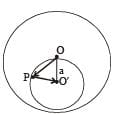Let us consider a uniformly charged solid sphere without any cavity. Let the charge per unit volume be σ and O be the centre of the sphere. Let us consider a uniformly charged sphere of negative charged density s having its centre at O'. Also let OO' be equal to a.

Let us consider an arbitrary point P in the small sphere.
The electric field due to charge on big sphere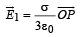Also the electric field due to small sphere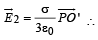The total electric field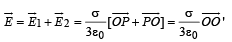Thus electric field will have a finite value which will be uniform.

Test: Single Correct MCQs: Electrostatics | JEE Advanced - Question 20

Positive and negative point charges of equal magnitude are kept at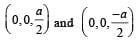respectively. The work done by the electric field when another positive point charge is moved from (–a, 0, 0) to (0, a, 0) is

Detailed Solution for Test: Single Correct MCQs: Electrostatics | JEE Advanced - Question 20

The charges make an electric dipole. A and B points lie on the equatorial plane of the dipole.
Therefore, potential at A = potential at B = 0

W = q (VA – VB) = q × 0 = 0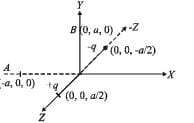Test: Single Correct MCQs: Electrostatics | JEE Advanced - Question 21

Consider a system of three charges q/3, q/3 and –2q/3 placed at points A, B and C, respectively, as shown in the figure.
Take O to be the centre of the circle of radius R and angle CAB = 60°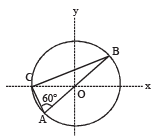Detailed Solution for Test: Single Correct MCQs: Electrostatics | JEE Advanced - Question 21

The electric field due to A and B at O are equal and opposite producing a resultant which is zero. The electric field at O due to C is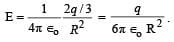∴ Option [A] is not correct. The electric potential at O is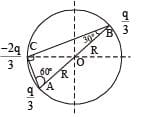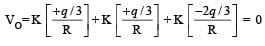∴ Option [D] is wrong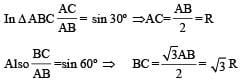Potential energy of the system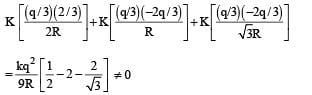∴ Option [B] is wrong Magnitude of force between B and C is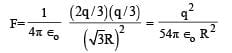Test: Single Correct MCQs: Electrostatics | JEE Advanced - Question 22

A parallel plate capacitor C with plates of unit area and separation d is filled with a liquid of dielectric constant K = 2. The level of liquid is d/3 initially. Suppose the liquid level decreases at a constant speed v, the time constant as a function of time t is –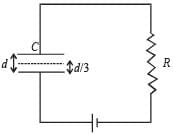Detailed Solution for Test: Single Correct MCQs: Electrostatics | JEE Advanced - Question 22

Let the level of liquid at an instant of time 't' be x. Then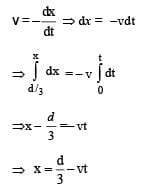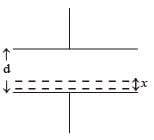Also the capacitance can be considered as an equivalent of two capacitances in series such that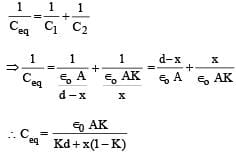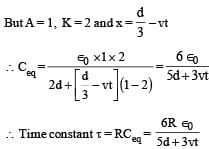Test: Single Correct MCQs: Electrostatics | JEE Advanced - Question 23

Three concentric metallic spherical shells of radii R,  2R, 3R, are given charges Q1, Q2, Q3, respectively. It is found that the surface charge densities on the outer surfaces of the shells are equal. Then, the ratio of the charges given to the shells, Q1 : Q2 : Q3, is

Detailed Solution for Test: Single Correct MCQs: Electrostatics | JEE Advanced - Question 23

The charges on the surfaces of the metallic spheres are shown in the diagram. It is given that the surface charge densities on the outer surfaces of the shells are equal.
Therefore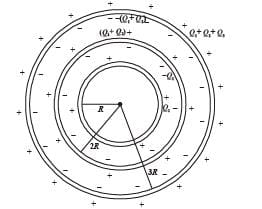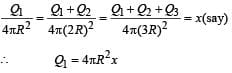Q1 + Q2 = 4π(2R)2k = 4[4πR2x]

⇒ Q2 = 4[4πR2 x] - Q1

= 4[4πR2x] -4πR2x = 3[4πR2x]
Also Q1 + Q2+ Q3 = 4π(3R)2 x = 9[4πR2x]
∴ Q3 = 9[4πR2x] - Q1 - Q2 = 9[4πR2x] - [4πR2x] -3[4πR2x] = 5[4πR2x]

⇒ Q1 : Q2 :Q3 = 1 :3 :5

Test: Single Correct MCQs: Electrostatics | JEE Advanced - Question 24

A disc of radius a / 4 having a uniformly distributed charge 6C is placed in the x - y plane with its centre at (–a / 2, 0, 0).
A rod of length a carrying a uniformly distributed charge 8C is placed on the x - axis from x = a /4 to x = 5a / 4. Two point charges – 7 C and 3 C are placed at (a / 4, – a /4, 0) and (– 3a /4, 3a / 4, 0), respectively. Consider a cubical surface formed by six surfaces x = ± a / 2, y = ± a / 2, z = ± a / 2. The electric flux through this cubical surface is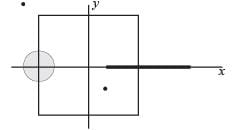Detailed Solution for Test: Single Correct MCQs: Electrostatics | JEE Advanced - Question 24

From the figure it is clear that the charge enclosed in the cubical surface is 3C + 2C – 7C = –2C. Therefore the electric flux through the cube is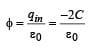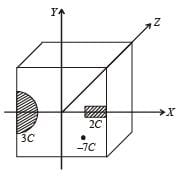Test: Single Correct MCQs: Electrostatics | JEE Advanced - Question 25

A uniformly charged thin spherical shell of radius R carries uniform surface charge density of σ per unit area. It is made of two hemispherical shells, held together by pressing them with force F (see figure). F is proportional to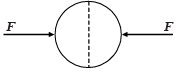Detailed Solution for Test: Single Correct MCQs: Electrostatics | JEE Advanced - Question 25

The electrostatic pressure at a point on the surface of a uniformly charged sphere  =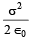∴ The force on a hemispherical shell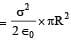Test: Single Correct MCQs: Electrostatics | JEE Advanced - Question 26

A tiny spherical oil drop carrying a net charge q is balanced in still air with a vertical uniform electric field of strength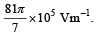When the field is switched off, the drop is observed to fall with terminal velocity 2 ×10–3 ms –1. Given g = 9.8 m s–2, viscosity of the air = 1.8 × 10–5 Ns m–2 and the density of oil = 900 kg m–3, the magnitude of q is

Detailed Solution for Test: Single Correct MCQs: Electrostatics | JEE Advanced - Question 26

When the electric field is on
Force due to electric field = weight

qE = mg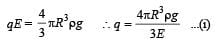When the electric field is switched off Weight = viscous drag force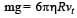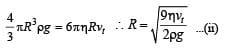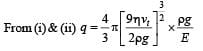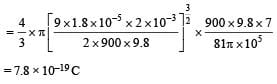Test: Single Correct MCQs: Electrostatics | JEE Advanced - Question 27

Consider an electric field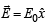where E0 is a constant.
The flux through the shaded area (as shown in the figure) due to this field is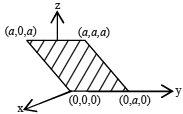Detailed Solution for Test: Single Correct MCQs: Electrostatics | JEE Advanced - Question 27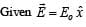This shows that the electric field acts along + x direction and is a constant. The area vector makes an angle of 45° with the electric field. Therefore the electric flux through the shaded portion whose area is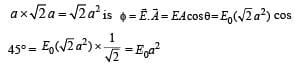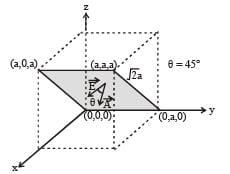Test: Single Correct MCQs: Electrostatics | JEE Advanced - Question 28

A 2 μF capacitor is charged as shown in the figure. The percentage of its stored energy dissipated after the switch S is turned to position 2 is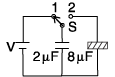Detailed Solution for Test: Single Correct MCQs: Electrostatics | JEE Advanced - Question 28

When S and 1 are connected

The 2μF capacitor gets charged. The potential difference across its plates will be V.
The potential energy stored in 2 μF capacitor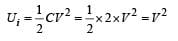When S and 2 are connected The 8mF capacitor also gets charged. During this charging process current flows in the wire and some amount of energy is dissipated as heat. The energy loss is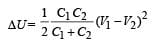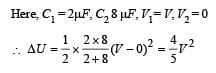The percentage of the energy dissipated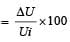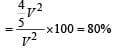Test: Single Correct MCQs: Electrostatics | JEE Advanced - Question 29

Which of the field patterns given below is valid for electric field as well as for magnetic field?

Detailed Solution for Test: Single Correct MCQs: Electrostatics | JEE Advanced - Question 29

The pattern of field lines shown in option (c) is correct because
(a) a current carrying toroid produces magnetic field lines of such pattern
(b) a changing magnetic field with respect to time in a region perpendicular to the paper produces induced electric field lines of such pattern.

Test: Single Correct MCQs: Electrostatics | JEE Advanced - Question 30

A wooden block performs SHM on a frictionless surface with frequency, v0. The block carries a charge +Q on its surface. If now a uniform electric field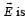switched-on as shown, then the SHM of the block will be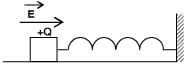Detailed Solution for Test: Single Correct MCQs: Electrostatics | JEE Advanced - Question 30

The frequency of SHM performed by wooden block is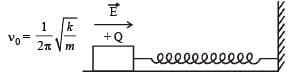when electric field is switched on, the value of k and m is not affected and therefore the frequency of SHM remains the same. But as an external force QE starts acting on the block towards right, the mean position of SHM shifts towards right by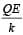correct option is (a).
Note : In SHM if a constant additional force is applied then it only shift the quilibrium position and does not change the frequency fo SHM.

Test: Single Correct MCQs: Electrostatics | JEE Advanced - Question 31

Two large vertical an d parallel metal plates having a separation of 1 cm are connected to a DC voltage source of potential difference X. A proton is released at rest midway between the two plates. It is found to move at 45° to the vertical JUST after release. Then X is nearly

Detailed Solution for Test: Single Correct MCQs: Electrostatics | JEE Advanced - Question 31

The two forces acting on the proton just after the release are shown in the figure. In this situation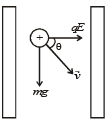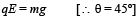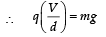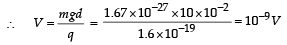Test: Single Correct MCQs: Electrostatics | JEE Advanced - Question 32

Consider a thin spherical shell of radius R with centre at the origin, carrying uniform positive surface charge density. The variation of the magnitude of the electric field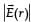and the electric potential V(r) with the distance r from the centre, is best represented by which graph?

Detailed Solution for Test: Single Correct MCQs: Electrostatics | JEE Advanced - Question 32

For a thin uniformly positive charged spherical shell

(i) Inside the shell at any point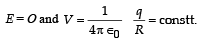where q = charge on sphere
R = Radius of sphere

(ii) Outside the shell at any point at any distance r from the centre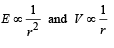Test: Single Correct MCQs: Electrostatics | JEE Advanced - Question 33

In the given circuit, a charge of +80 μC is given to the upper plate of the 4 μF capacitor. Then in the steady state, the charge on the upper plate of the 3 μF capacitor is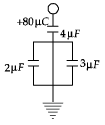Detailed Solution for Test: Single Correct MCQs: Electrostatics | JEE Advanced - Question 33

The total charge on plate A will be – 80 µC. If qB and qC be the charges on plate B and C then

qB + qC = 80 µC ...(1)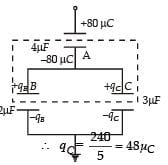Also 2µF and 3µF capacitors are in parallel. Therefore,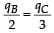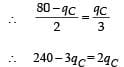This charge will obviously be positive.

Test: Single Correct MCQs: Electrostatics | JEE Advanced - Question 34

Charges Q, 2Q and 4Q are uniformly distributed in three dielectric solid spheres 1, 2 and 3 of radii R/2, R and 2R respectively, as shown in figure. If magnitude of the electric fields at point P at a distance R from the centre of sphere 1, 2 and 3 are E1, E2 and E3 respectively, then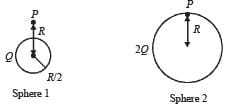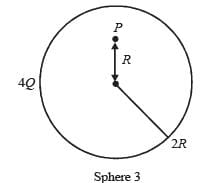Detailed Solution for Test: Single Correct MCQs: Electrostatics | JEE Advanced - Question 34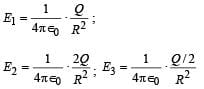Clearly E2 > E1 > E3 where Q/2 is the charge enclosed in a sphere of radius R concentric with the given sphere.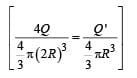## Physics For JEE

252 videos|559 docs|257 tests
 Use Code STAYHOME200 and get INR 200 additional OFF Use Coupon Code
Information about Test: Single Correct MCQs: Electrostatics | JEE Advanced Page
In this test you can find the Exam questions for Test: Single Correct MCQs: Electrostatics | JEE Advanced solved & explained in the simplest way possible. Besides giving Questions and answers for Test: Single Correct MCQs: Electrostatics | JEE Advanced, EduRev gives you an ample number of Online tests for practice

## Physics For JEE

252 videos|559 docs|257 tests